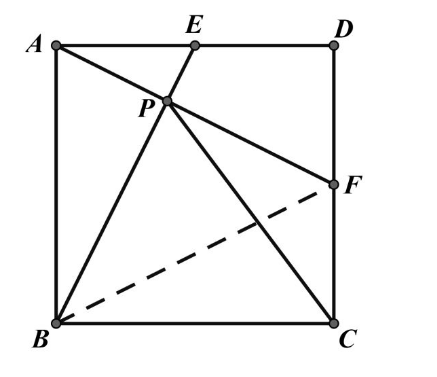【答案】 简证：易知 $\triangle A B E \cong \triangle D A F$
$B E \perp A F, \therefore B 、 C 、 F 、 P$ 四点共圆
\begin{aligned} & \angle B P C=\angle B F C \\ & \angle P B C=\angle B E A \\ & \text { 而 } \angle B E A=\angle B F C \\ & \therefore \angle B P C=\angle P B C \\ & \therefore P C=B C \text { 。 } \end{aligned}